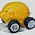## Tuesday, May 26, 2015

### Dynamics of the savings rate and Solow + IS-LM

Hello! I'm back from a short vacation and slowly getting to the comments.

As I mentioned here, there might be a bit more to the information equilibrium picture of the Solow model than just the basic mechanics -- in particular I pointed out we might be able to figure out some dynamics of the savings rate relative to demand shocks.

In the previous post, we built the model:

$$Y \rightarrow K \rightarrow I$$

Where $Y$ is output, $K$ is capital and $I$ is investment. Since information equilibrium (IE) is an equivalence relation, we have the model:

$$p: Y \rightarrow I$$

with abstract price $p$ which was described here (except using the symbol $N$ instead of $Y$) in the context of the IS-LM model. If we write down the differential equation resulting from that IE model

$$\text{(1) }\;\; p = \frac{dY}{dI} = \frac{1}{\eta} \; \frac{Y}{I}$$

There are a few of things we can glean from this ...

I. General equilibrium

We can solve equation (1) under general equilibrium giving us $Y \sim I^{1/\eta}$. Empirically, we have $\eta \simeq 1$:

Combining that with the results from the Solow model, we have

$$Y \sim K^{\alpha} \; \text{,} \; K \sim I^{\sigma} \; \text{and} \; Y \sim I$$

which tells us that $\alpha \simeq 1/\sigma$ -- one of the conditions that gave us the original Solow model.

II. Partial equilibrium

Since $Y \rightarrow I$ we have a supply and demand relationship between output and investment in partial equilibrium. We can use equation (1) and $\eta = 1$ to write

$$I = (1/p) Y \equiv s Y$$

Where we have defined the saving rate as $s \equiv 1/(p \eta)$ to be (the inverse of) the abstract price $p$ in the investment market. The supply and demand diagram (including an aggregate demand shock) looks like this:

A shock to aggregate demand would be associated in a fall in the abstract price and thus a rise in the savings rate. There is some evidence of this in the empirical data:

Overall, you don't always have pure supply or demand shocks, so there might be some deviations from a pure demand shock view. In particular, a "supply shock" (investment shock) should lead to a fall in the savings rate.

III. Interest rates

If we update the model here (i.e. the IS-LM model mentioned above) to include the more recent interest rate ($r$) model written in terms of investment and the money supply/base money:

$$(r \rightarrow p_{m}) : I \rightarrow M$$

where $p_{m}$ is the abstract price of money (which is in IE with the interest rate), we have a pretty complete model of economic growth that combines the Solow model with the IS-LM model. The interest rate joins the already empirically accurate production function:

Since I inevitably get questions about causality, it is important to note that these are all IE relationships therefore all relationships are effectively causal in either direction. However it is also important to note that the direct impact of $M$ on $Y$ is neglected in the above treatment (including the interest rates) -- and the direct impact changes depending on the information transfer index in the price level model.

Summary

A full summary of the Solow + IS-LM model in terms of IE relationships is:

$$Y \rightarrow K \rightarrow I \; \text{,} \; K \rightarrow D$$

$$Y \rightarrow L$$

$$1/s : Y \rightarrow I$$

$$(r \rightarrow p_{m}) : I \rightarrow M$$

Update 5/27/2015: Forgot first graph; corrected.

1.Lots of plucking in your interest rate model

1.Maybe I should say plucking away from your interest rate model?

2.But only in the new era of financial crises....Maybe some downward bias in the inflationary 70s...interesting so both inflationary episodes and financial herding are not entropy maximal...

3.This comment has been removed by the author.